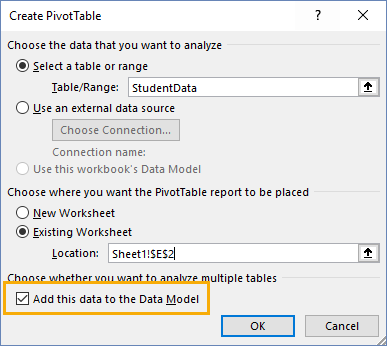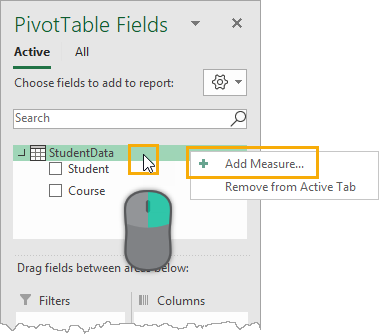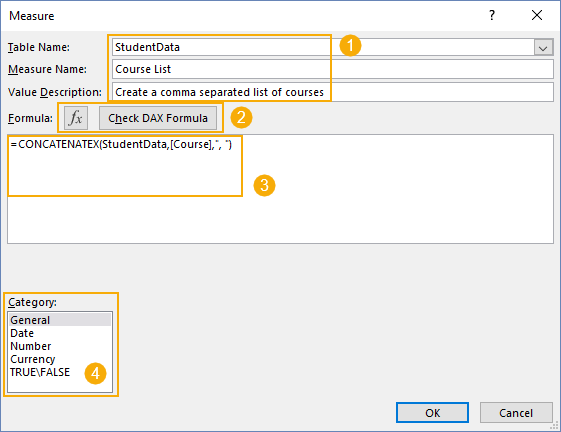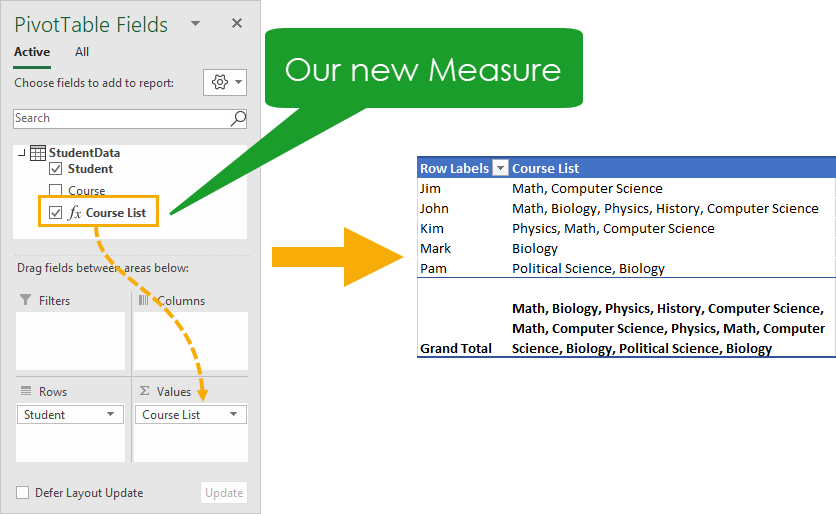I got a rather interesting question from someone who attended one of my pivot table webinars. They were wondering if they could have text values in the Values area of a pivot table?

This is usually the area where we summarize fields by various different aggregation methods like taking the sum, average, minimum, maximum or standard deviation.But the thing is, these aggregation methods require numeric data!

Is there any way to summarize text based data that will return text as the result?

The answer is yes, but we will need to use the data model and DAX formulas to do this. Traditional pivot tables do not have this functionality.

Also, we will need to be a PC user with Excel 2013 (or later) and Office 365. Sorry, but these modern features aren’t available in the Mac versions yet.

The ProblemHere we’ve got a list of students along with the courses they are enrolled in. A student can have multiple rows of data when they are enrolled in multiple courses.

Can we summarize this data with a pivot table so that we just display each student once and then show a comma separated list of their courses?

## Insert A Pivot Table

First, we will need to insert a pivot table. This is done in the usual manner.

Select a cell inside the data ➜ go to the Insert tab ➜ then press the Pivot Table button.

In order to use DAX formulas, we will need to select the Add this to the Data Model option.

With traditional pivot tables, we don’t need to define any calculations. They come predefined with basic sum, count, average, minimum, maximum, standard deviation and variance calculations.

With the data model, we get access to a whole new world of possible calculations using DAX formulas. These are created by adding Measures. We can create just about any calculation we can imagine with these.To add a Measure, select the pivot table ➜ right click on the table of data found in the PivotTable Fields window ➜ choose Add Measure from the menu.

This will open the Measure dialog box where we can create our DAX formulas.

## ConcatenateX FunctionIn the Measure dialog box:

1. We need to select the table to which to attach our measure, give the measure a name and description.
2. We can explore the available DAX formulas using the Insert Function menu and also check the validity of any formula we write.
3. We can write our formula in the DAX formula editor.
4. We can assign formatting to the measure.

To create a measure that aggregates text into a comma separated list, we’re going to use the ConcatenateX DAX function.

``=CONCATENATEX(StudentData,[Course],", ")``

We need to write the above formula into the DAX formula editor and then we can create the new measure by pressing the OK button.

This will take the Course field from the StudentData table and concatenate its values together with a comma and space character as a delimiter.

## Using Our New MeasureThis new measure will appear listed in the PivotTable Fields window with all the other fields and we can use this new measure just like any of the other fields.

Measures can easily be identified from the data fields by the fx icon in from of the measure name.

We can click and drag the Course List measure into the Values area of our pivot table and this will produce a comma separated list of a students courses.

## Conclusions

Pivot tables have been a feature in Excel for a long time and they can do a lot of great useful calculations.

They are limited though, especially when it comes to displaying text values in the Values area.

With the data model we get many new calculation options that regular pivot tables just don’t have, including concatenating text values to display in the Values area. This is definitely a feature worth exploring when regular pivot tables just won’t cut it.